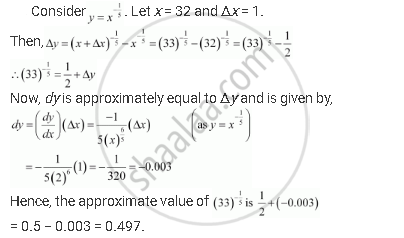Share

# Using Differentials, Find the Approximate Value of Each of the Following. (33)^(1/5) - CBSE (Science) Class 12 - Mathematics

#### Question

Using differentials, find the approximate value of each of the following.

(33)^(1/5)

#### SolutionIs there an error in this question or solution?

#### APPEARS IN

NCERT Solution for Mathematics Textbook for Class 12 (2018 to Current)
Chapter 6: Application of Derivatives
Q: 1.2 | Page no. 242

#### Video TutorialsVIEW ALL 

Solution Using Differentials, Find the Approximate Value of Each of the Following. (33)^(1/5) Concept: Approximations.
S